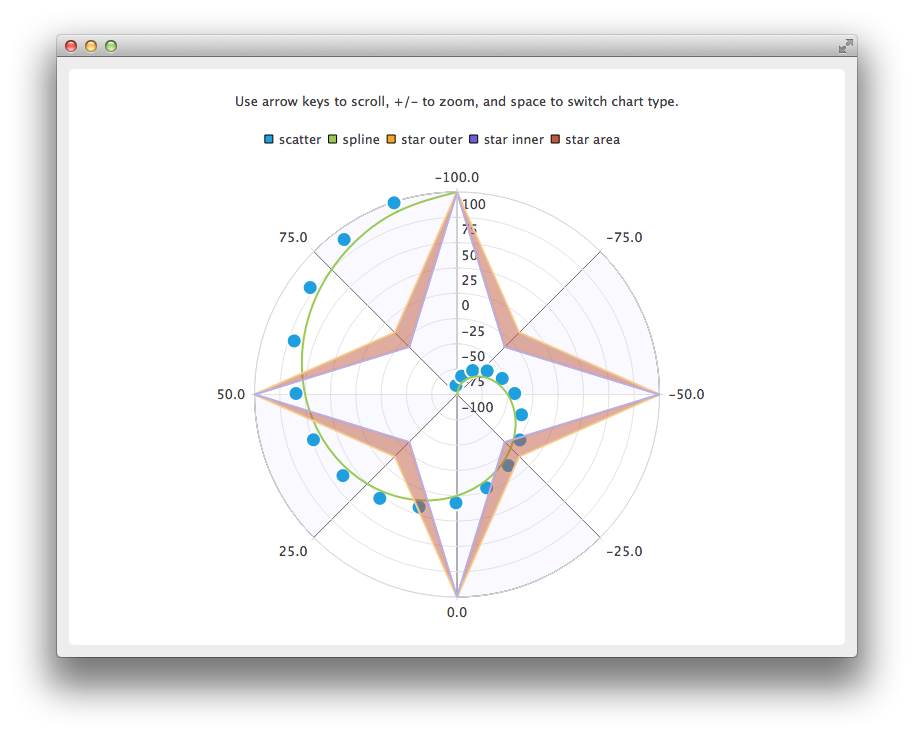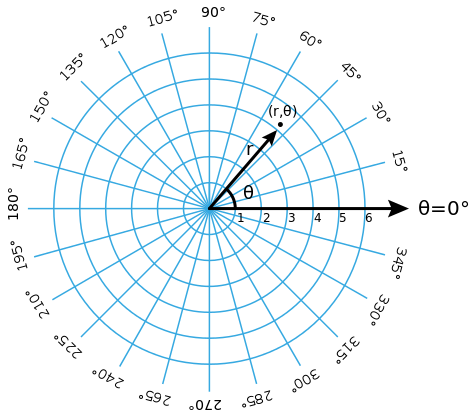# QPolarChart change axis orientation

• My goal is to use QPolarChart from Qt Charts library, but with different orientation of polar axes.
I.e. axes r (distance) pointing right and \theta (angle) axis getting positive values counter-clock-wise.

In detail: Official Qt examples show QPolarChart as follows (see Picture below). I.e. the r axis orientated as bottom-top (i.e. as Y axis, pointing to top) and \theta axis getting positive values when rotating clock-wise (i.e. positive values are rotated clock-wise).My goal though is to have QPolarChart looking like on the picture below, which means (1) the r axis points right (i.e. orientated as X axis, instead of pointing top as by default), (2) positive values of \theta angle are counted counter-clock-wise (instead of clock-wise for positive by default):For this I would need either to rotate the plot 90 degrees around the center clock-wise, and then "mirror" the plot (i.e. rotate a plot around X axis 180 degrees), or maybe some elegant solution exists. Rotating the whole chart can be done, but it also rotates chart title, and everything else. When rotating my goal would be to rotate "axes" and plot only. Cannot find anything related to QPolarChart in this respect..